Next: Small-Perturbation Theory Up: Two-Dimensional Compressible Inviscid Flow Previous: Crocco's Theorem

# Homenergic Homentropic Flow

Consider a steady, two-dimensional, homenergic, homentropic flow pattern, in the absence of body forces. Suppose that all quantities are independent of the Cartesian coordinate, and that the flow velocity,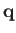, is confined to the-plane. Let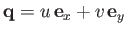. Equations (14.25), (14.30), and (14.31) reduce to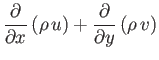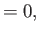(15.92)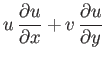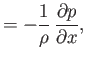(15.93)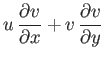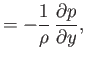(15.94)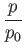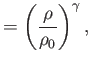(15.95)

where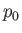and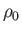are the uniform stagnation pressure and density, respectively. (Note thatandmust be uniform because the stagnation specific entropy,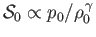, and the stagnation temperature,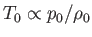, are both uniform.) Equation (15.95) implies that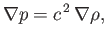(15.96)

where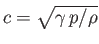is the sound speed. Hence, Equations (15.92)-(15.94) yield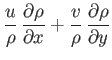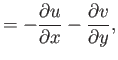(15.97)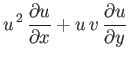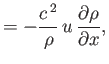(15.98)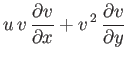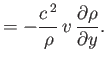(15.99)

Summing the previous two equations, and then making use of Equation (15.97), we obtain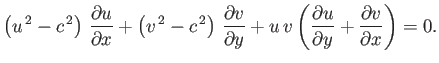(15.100)

Finally, given that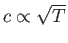, Equation (14.58) implies that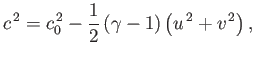(15.101)

where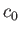is the stagnation sound speed.Next: Small-Perturbation Theory Up: Two-Dimensional Compressible Inviscid Flow Previous: Crocco's Theorem
Richard Fitzpatrick 2016-03-31